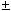Online Digital Electronics Test - Digital Electronics Test 6Loading Test...

Instruction:

• This is a FREE online test. DO NOT pay money to anyone to attend this test.
• Total number of questions : 20.
• Time alloted : 30 minutes.
• Each question carry 1 mark, no negative marks.
• DO NOT refresh the page.
• All the best :-).

1.

The horizontal axis of an oscilloscope is normally calibrated in units of ________.

A.
 voltageB.
 currentC.
 timeD.
 frequencyCorrect Answer: Option C

Learn more problems on : Digital Concepts

2.

Which of the following combinations cannot be combined into K-map groups?

A.
 Corners in the same rowB.
 Corners in the same columnC.
 Diagonal cornersD.
 Overlapping combinationsCorrect Answer: Option C

Learn more problems on : Combinational Logic Circuits

3.

If an active-HIGH S-R latch has a 0 on the S input and a 1 on the R input and then the R input goes to 0, the latch will be ________.

A.
 SETB.
 RESETC.
 clearD.
 invalidCorrect Answer: Option B

4.

Which segments of a seven-segment display would be required to be active to display the decimal digit 2?

A.
 a, b, d, e, and gB.
 a, b, c, d, and gC.
 a, c, d, f, and gD.
 a, b, c, d, e, and fCorrect Answer: Option A

5.

The address-decoding scheme for a 16K-byte EPROM memory system requires a 1-to-8-address decoder when 4K × 8 memory is used.

A.
 TrueB.
 FalseCorrect Answer: Option A

Learn more problems on : Memory and Storage

6.

When the term RAM is used with semiconductor memories, it usually means ________ as opposed to ROM.

A.
 Random-Access MemoryB.
 Read/Write Memory (RWM)C.
 flash memoryD.
 temporary storageCorrect Answer: Option B

Learn more problems on : Memory and Storage

7.

How many pins are in an EDF10K70 package?

A.
 70B.
 140C.
 240D.
 532Correct Answer: Option C

Learn more problems on : Programmable Logic Device

8.

How many macrocells are in a MAX700S LAB?

A.
 8B.
 16C.
 32D.
 64Correct Answer: Option B

Learn more problems on : Programmable Logic Device

9.

In a digital reproduction of an analog curve, accuracy can be increased by ________.

A.
 sampling the curve more oftenB.
 sampling the curve less oftenC.
 decreasing the number of bits used to represent each sampled valueD.
 all of the aboveCorrect Answer: Option A

Learn more problems on : Digital Signal Processing

10.

Settling time is normally defined as the time it takes a DAC to settle within ________.

A.1/8 LSB of its final value when a change occurs in the input codeB.1/4 LSB of its final value when a change occurs in the input codeC.1/2 LSB of its final value when a change occurs in the input codeD.
 1 LSB of its final value when a change occurs in the input codeCorrect Answer: Option C

Learn more problems on : Digital Signal Processing

11.

ECL gates are noted for their high frequency capability and small output voltage swing.

A.
 TrueB.
 FalseCorrect Answer: Option A

Learn more problems on : Integrated-Circuit Logic Families

12.

Three characteristics of op amps make them almost ideal amplifiers: very high input impedance, very low impedance, and ________.

A.
 very high voltage gainB.
 unlimited bandwidthC.
 a low slew rateD.
 very high current gainCorrect Answer: Option A

Learn more problems on : Interfacing to the Analog World

13.

D/A conversion is the process of taking a voltage or current and converting it to a digital code.

A.
 TrueB.
 FalseCorrect Answer: Option B

Learn more problems on : Interfacing to the Analog World

14.

The serial format for transmitting binary information uses:

A.
 a single conductorB.
 multiple conductorsC.
 infrared technologyD.
 fiber-opticCorrect Answer: Option A

Learn more problems on : Signals and Switches

15.

What is the minimum voltage required before a diode will allow current to flow between the cathode and the anode?

A.
 0.7 VB.
 0.07 VC.
 4.3 VD.
 0.2 VCorrect Answer: Option A

Learn more problems on : Signals and Switches

16.

In a priority encoder, the input with the highest priority is represented on the output.

A.
 TrueB.
 FalseCorrect Answer: Option A

Learn more problems on : Code Converters and Multiplexers

17.

Ten TTL loads per TTL driver is known as:

A.
 noise immunityB.
 fan-outC.
 power dissipationD.
 propagation delayCorrect Answer: Option B

Learn more problems on : Logic Families and Their Characteristics

18.

Look up the propagation delay from the clock to the output for the 7476. Are the HIGH-to-LOW and LOW-to-HIGH propagation delays the same?

A.
 yesB.
 no, tPLH = 25 ns, tPHL = 40 nsC.
 no, tPLH = 40 ns, tPHL = 25 nsD.
 no, tPHL = 25 ns, tPLH = 40 nsCorrect Answer: Option B

Learn more problems on : Digital Design

19.

Which of the following flip-flop timing parameters indicates the time it takes a Q output to respond to a Cp input?

A.
 ts, thB.
 tPHL, tPLHC.
 tw (L), tw (H)D.
 fmaxCorrect Answer: Option B

Learn more problems on : Digital Design

20.

A monostable 555 timer has the following number of stable states:

A.
 0B.
 1C.
 2D.
 3Correct Answer: Option B

Learn more problems on : Multivibrators and 555 Timer